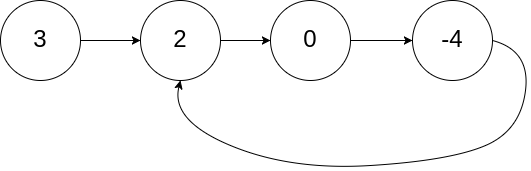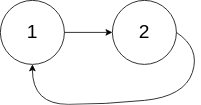# LeetCode141. Linked List Cycle（链表 / 模拟）

## LeetCode141. Linked List Cycle（链表 / 模拟）

• Easy
• Accepted：385,781
• Submissions：1,059,839

Given a linked list, determine if it has a cycle in it.

To represent a cycle in the given linked list, we use an integer pos which represents the position (0-indexed) in the linked list where tail connects to. If pos is -1, then there is no cycle in the linked list.

Example 1:

Input: head = [3,2,0,-4], pos = 1
Output: true
Explanation: There is a cycle in the linked list, where tail connects to the second node.Example 2:

Input: head = [1,2], pos = 0
Output: true
Explanation: There is a cycle in the linked list, where tail connects to the first node.Example 3:

Input: head = , pos = -1
Output: false
Explanation: There is no cycle in the linked list.Can you solve it using O(1) (i.e. constant) memory?

### 题解

• 哈希的话，就相当于判断数组中是否有相同的数，我们判断的是链表中是否存在相同的结点，使用unordered_set存入链表结点，如果链表存在环，那么最后一个结点肯定会指向之前出现过的结点，时间复杂度$O(n)​$，空间复杂度$O(n)​$
• 使用快慢指针slow和fast，slow开始指向head，fast开始指向head->next，然后slow每次往前走一步，fast每次往前走两步，如果链表中存在环，那么slow和fast后面肯定会相遇，注意判断结点为空的情况，时间复杂度$O(n)$，空间复杂度$O(1)​$

### 代码

• Runtime: 12 ms, faster than 99.37% of C++ online submissions for Linked List Cycle.
• Memory Usage: 9.7 MB, less than 64.15% of C++ online submissions for Linked List Cycle.
class Solution {
public:
return false;
while (slow != fast)
{
if (fast == NULL || fast->next == NULL)
return false;
slow = slow->next;
fast = fast->next->next;
}
return true;
}
};
• Runtime: 28 ms, faster than 5.32% of C++ online submissions for Linked List Cycle.
• Memory Usage: 11.9 MB, less than 10.38% of C++ online submissions for Linked List Cycle.
class Solution {
public:
return false;
unordered_set<ListNode* > uset;
{
};